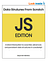# Combinatorics — Counting dice combinations using JavaScript

Quite frequently I got asked questions like:

“How can I get all possible combinations for a given dice roll?”

“How many combinations add up to 7 in a pair of dice?”

“Is it possible to use a recursive algorithm to count all dice combinations?”

To answer these questions (and variants of them that involve coins, cards decks, and whatnot) we can use combinatorics, a branch of mathematics that deals with combinations of objects belonging to a finite set of elements.

While I’m not an expert in the subject, in this post I’ll try to show you how to solve combinatorics problems using JavaScript.

But before jumping into the code, let’s disambiguate combinations from permutations. Two separate concepts that sometimes people use interchangeably.

Permutations are all possible combinations of a given list of ordered values. For instance, while calculating all possible permutations for two 6 sided dice, the pairs {1, 6} and {6,1} are considered to be different. The order matters.

When counting combinations, the pairs {1, 6} and {6, 1} are considered to be the same. So, as it happens when we are working with sets, the order doesn’t matter.

To hit two birds with one stone, we are not going to use loops. Performance wise, using recursive functions instead of loops is not the most efficient way to go (at least not in JavaScript) but since most people were asking for a recursive solution, that’s the route that we are going to take.

First off, let’s create a function that gives us all unique combinations for the given number of dice. Once we are done with that, we’ll all add a filter to include only those combinations that add up to some particular number.

`const SIDES = 6;function append(src, num) {  res = Array.from(src);  res.push(num);  return res;}function diceCombinations(dc, acc, side, res) {  if (dc < 1 || side >= (SIDES + 1))    return;  if (dc == 1) {    let set = append(acc, side);    res.push(set);  }  else {    diceCombinations(dc — 1, append(acc, side), side, res);  }  return diceCombinations(dc, acc, side + 1, res);}`

For 2 dice, the previous function returns 21 unique combinations.

Sure.

No, wait!

6 sides per die * 2 dice = 6**2 = 36 possible combinations!

Generally speaking, your math is right; But keep in mind that we are counting unique sets of values; In this setting, the pairs {1, 2} and {2, 1} are considered to be the same. And that’s why the correct answer is 21.

If we use 3 dice, it’ll be 56, and so on…

(If you are interested in the theory/math behind combinatorics, I recommend you to take a look at this short article. No worries, you don’t need a Ph.D. to understand it :))

Now that we know that our function counts the right number of combinations, let’s add a filter to include only those combinations that add up to a given number. For instance, how many unique combinations add up to 7 if we are using 2 dice, and stuff like that.

`function diceCombinations(dc, acc, side, target, res) {  if (dc < 1 || side >= (SIDES + 1))    return;   if (dc == 1) {     let set = append(acc, side);     if (sumSet(set, 0) == target)       res.push(set);     }     else {       diceCombinations(         dc — 1, append(acc, side), side, target, res);  }  return diceCombinations(dc, acc, side + 1, target, res);}````

I also added a helper function to sum the elements of the set. (Just for fun, I made it recursive, too.)

`// Sums the value of all members in the set.function sumSet(set, idx) {  if (idx >= set.length)    return 0;  return set[idx] + sumSet(set, idx + 1);}`

Now our function allows us to answer:

“How many combinations add up to 7 while rolling a pair of dice?”

`{1, 6} {2, 5} {3, 4}`

“What if we use 3?”

`{1, 1, 5} {1, 2, 4} {1, 3, 3} {2, 2, 3}`

So, that’s it for this short post. I hope it helps you to learn a bit about combinatorics.

Thanks for reading!

See you next time.

Before you go. If you are getting started with algorithms and data structures, you might want to take a look at my ebook “Data Structures From Scratch — JS Edition” (It free for Kindle Unlimited members.)Data Structures from scratch — JS Edition

Also, don’t forget to clap if you like this post!!

Written by

## amiralles

#### Concise programming articles for those who code

Welcome to a place where words matter. On Medium, smart voices and original ideas take center stage - with no ads in sight. Watch
Follow all the topics you care about, and we’ll deliver the best stories for you to your homepage and inbox. Explore
Get unlimited access to the best stories on Medium — and support writers while you’re at it. Just \$5/month. Upgrade